NCERT Exemplar Class 10 Science Chapter 10: Light – Reflection and Refraction. NCERT Exemplar Solutions for Class 10 Science Chapter 10 Light – Reflection and Refraction prepare students for their Class 10 exams thoroughly.

Science problems and solutions for the Class 10 pdf are provided here which are similar to the questions being asked in the previous year’s board.

## NCERT Exemplar Class 10 Science Chapter 10: Light – Reflection and Refraction

Class 10 : Science Chapter 10 solutions. Complete Class 10 Science Chapter 10 Notes.

Multiple Choice Questions

1. hich of the following can make a parallel beam of light when light from a point source is incident on it?
• (a) Concave mirror as well as convex lens
• (b) Convex mirror as well as concave lens
• (c) Two plane mirrors placed at 90° to each other
• (d) Concave mirror as well as concave lens
2. A 10 mm long awl pin is placed vertically in front of a concave mirror. A 5 mm long image of the awl pin is formed at 30 cm in front of the mirror. The focal length of this mirror is
• (a) – 30 cm
• (b) – 20 cm
• (c) – 40 cm
• (d) – 60 cm
3. Under which of the following conditions a concave mirror can form an image larger than the actual object?
• (a) When the object is kept at a distance equal to its radius of curvature
• (b) When object is kept at a distance less than its focal length
• (c) When object is placed between the focus and centre of curvature
• (d) When object is kept at a distance greater than its radius of curvature
4. Figure 10.1 shows a ray of light as it travels from medium A to medium B. Refractive index of the medium B relative to medium A is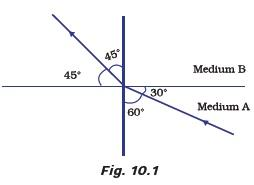• (a) √3 / √2
• (b) √2 / √3
• (c) 1 / √2
• (d) √2
5. A light ray enters from medium A to medium B as shown in Figure 10.2. The refractive index of medium B relative to A will be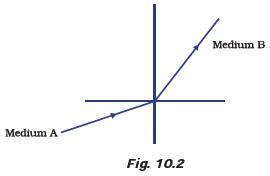• (a) greater than unity
• (b) less than unity
• (c) equal to unity
• (d) zero
6. Beams of light are incident through the holes A and B and emerge out of box through the holes C and D respectively as shown in the Figure 10.3. Which of the following could be inside the box?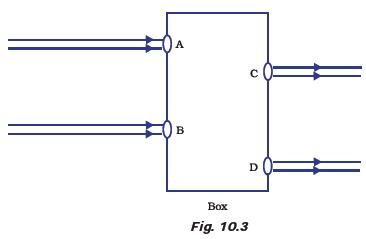• (a) A rectangular glass slab
• (b) A convex lens
• (c) A concave lens
• (d) A prism
7. A beam of light is incident through the holes on side A and emerges out of the holes on the other face of the box as shown in the Figure 10.4. Which of the following could be inside the box?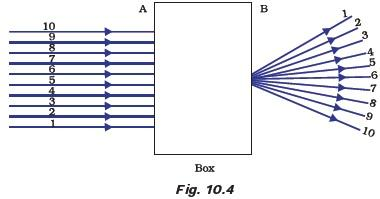• (a) Concave lens
• (b) Rectangular glass slab
• (c) Prism
• (d) Convex lens
8. Which of the following statements is true?
• (a) A convex lens has 4 dioptre power having a focal length 0.25 m
• (b) A convex lens has –4 dioptre power having a focal length 0.25 m
• (c) A concave lens has 4 dioptre power having a focal length 0.25 m
• (d) A concave lens has –4 dioptre power having a focal length 0.25 m
9. Magnification produced by a rear view mirror fitted in vehicles
• (a) is less than one
• (b) is more than one
• (c) is equal to one
• (d) can be more than or less than one depending upon the position of the object in front of it
10. Rays from Sun converge at a point 15 cm in front of a concave mirror. Where should an object be placed so that size of its image is equal to the size of the object?
• (a) 15 cm in front of the mirror
• (b) 30 cm in front of the mirror
• (c) between 15 cm and 30 cm in front of the mirror
• (d) more than 30 cm in front of the mirror
11. A full length image of a distant tall building can definitely be seen by using
• (a) a concave mirror
• (b) a convex mirror
• (c) a plane mirror
• (d) both concave as well as plane mirror
12. In torches, search lights and headlights of vehicles the bulb is placed
• (a) between the pole and the focus of the reflector
• (b) very near to the focus of the reflector
• (c) between the focus and centre of curvature of the reflector
• (d) at the centre of curvature of the reflector
13. The laws of reflection hold good for
• (a) plane mirror only
• (b) concave mirror only
• (c) convex mirror only
• (d) all mirrors irrespective of their shape
14. The path of a ray of light coming from air passing through a rectangular glass slab traced by four students are shown as A, B, C and D in Figure 10.5. Which one of them is correct?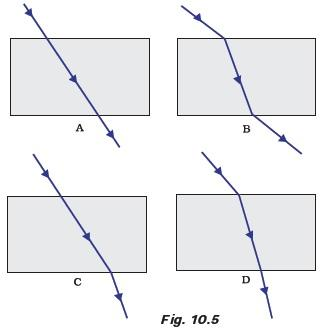15. You are given water, mustard oil, glycerine and kerosene. In which of these media a ray of light incident obliquely at same angle would bend the most?
• (a) Kerosene
• (b) Water
• (c) Mustard oil
• (d) Glycerine
16. Which of the following ray diagrams is correct for the ray of light incident on a concave mirror as shown in Figure 10.6?• (a) Fig. A
• (b) Fig. B
• (c) Fig. C
• (d) Fig. D
17. Which of the following ray diagrams is correct for the ray of light incident on a lens shown in Fig. 10.7?• (a) Fig. A.
• (b) Fig. B.
• (c) Fig. C.
• (d) Fig. D.
18. A child is standing in front of a magic mirror. She finds the image of her head bigger, the middle portion of her body of the same size and that of the legs smaller. The following is the order of combinations for the magic mirror from the top.
• (a) Plane, convex and concave
• (b) Convex, concave and plane
• (c) Concave, plane and convex
• (d) Convex, plane and concave
19. In which of the following, the image of an object placed at infinity will be highly diminished and point sized?
• (a) Concave mirror only
• (b) Convex mirror only
• (c) Convex lens only
• (d) Concave mirror, convex mirror, concave lens and convex lens

1. Identify the device used as a spherical mirror or lens in following cases, when the image formed is virtual and erect in each case.
• (a) Object is placed between device and its focus, image formed is enlarged and behind it.
• (b) Object is placed between the focus and device, image formed is enlarged and on the same side as that of the object.
• (c) Object is placed between infinity and device, image formed is diminished and between focus and optical centre on the same side as that of the object.
• (d) Object is placed between infinity and device, image formed is diminished and between pole and focus, behind it.
2. Why does a light ray incident on a rectangular glass slab immersed in any medium emerges parallel to itself? Explain using a diagram.
3. A pencil when dipped in water in a glass tumbler appears to be bent at the interface of air and water. Will the pencil appear to be bent to the same extent, if instead of water we use liquids like, kerosene or turpentine. Support your answer with reason.
4. How is the refractive index of a medium related to the speed of light? Obtain an expression for refractive index of a medium with respect to another in terms of speed of light in these two media?
5. Refractive index of diamond with respect to glass is 1.6 and absolute refractive index of glass is 1.5. Find out the absolute refractive index of diamond.
6. A convex lens of focal length 20 cm can produce a magnified virtual as well as real image. Is this a correct statement? If yes, where shall the object be placed in each case for obtaining these images?
7. Sudha finds out that the sharp image of the window pane of her science laboratory is formed at a distance of 15 cm from the lens. She now tries to focus the building visible to her outside the window instead of the window pane without disturbing the lens. In which direction will she move the screen to obtain a sharp image of the
building? What is the approximate focal length of this lens?
8. How are power and focal length of a lens related? You are provided with two lenses of focal length 20 cm and 40 cm respectively. Which lens will you use to obtain more convergent light?
9. Under what condition in an arrangement of two plane mirrors, incident ray and reflected ray will always be parallel to each other, whatever may be angle of incidence. Show the same with the help of diagram.
10. Draw a ray diagram showing the path of rays of light when it enters with oblique incidence
• (i) from air into water;
• (ii) from water into air.

1. Draw ray diagrams showing the image formation by a concave mirror when an object is placed
• (a) between pole and focus of the mirror
• (b) between focus and centre of curvature of the mirror
• (c) at centre of curvature of the mirror
• (d) a little beyond centre of curvature of the mirror
• (e) at infinity
2. Draw ray diagrams showing the image formation by a convex lens when an object is placed
• (a) between optical centre and focus of the lens
• (b) between focus and twice the focal length of the lens
• (c) at twice the focal length of the lens
• (d) at infinity
• (e) at the focus of the lens
3. Write laws of refraction. Explain the same with the help of ray diagram, when a ray of light passes through a rectangular glass slab.
4. Draw ray diagrams showing the image formation by a concave lens when an object is placed
• (a) at the focus of the lens
• (b) between focus and twice the focal length of the lens
• (c) beyond twice the focal length of the lens
5. Draw ray diagrams showing the image formation by a convex mirror when an object is placed
• (a) at infinity
• (b) at finite distance from the mirror
6. The image of a candle flame formed by a lens is obtained on a screen placed on the other side of the lens. If the image is three times the size of the flame and the distance between lens and image is 80 cm, at what distance should the candle be placed from the lens? What is the nature of the image at a distance of 80 cm and the lens?
7. Size of image of an object by a mirror having a focal length of 20 cm is observed to be reduced to 1/3rd of its size. At what distance the object has been placed from the mirror? What is the nature of the image and the mirror?
8. Define power of a lens. What is its unit? One student uses a lens of focal length 50 cm and another of –50 cm. What is the nature of the lens and its power used by each of them?
9. A student focussed the image of a candle flame on a white screen using a convex lens. He noted down the position of the candle screen and the lens as under
Position of candle = 12.0 cm
Position of convex lens = 50.0 cm
Position of the screen = 88.0 cm
• (i) What is the focal length of the convex lens?
• (ii) Where will the image be formed if he shifts the candle towards the lens at a position of 31.0 cm?
• (iii) What will be the nature of the image formed if he further shifts the candle towards the lens?
• (iv) Draw a ray diagram to show the formation of the image in case (iii) as said above.

## Answers to Multiple Choice Questions

Multiple Choice Questions